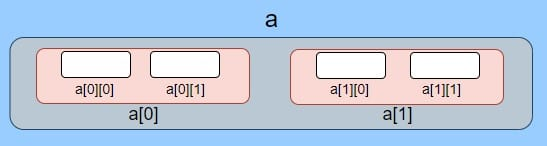# Multidimensional Arrays in Java

In this lesson, we will cover a multidimensional array in Java.

The arrays we have discussed so far have only one “dimension” – length.  Ordinary arrays are therefore often called one-dimensional arrays.

In Java, we have arrays that have two dimensions- length and height, or three dimensions – length, height and depth, and so on. That’s why these arrays are known as multidimensional arrays.

Multidimensional arrays in Java represent data in matrix form in which data get stored in rows and columns.## Declaring a multidimensional array in Java

We have three valid ways to declare a multidimensional array in Java.

```class Test {

int[][] array1;
int[][] array2;
int array3[][];
}
```

## Instantiation and Initialization of a Multidimensional Java array

```class Test {

int[][] array1 = new int; // 3 rows and 3 columns
int[][] array2 = {{1, 2}, {3, 4}}; // 2 rows and 2 columns
}
```

We can initialize this two-dimensional array by specifying how many rows and columns it will have, and we can also immediately add values/one-dimensional arrays to it.

Let’s see one example when we first initialize the array and then add elements:

```class Test {

public static void main(String[] args) {
int[][] array = new int; // 2 rows and 2 columns

// add elements to first array/row
array = 1;
array = 2;

// add elements to the second array/row
array = 3;
array = 4;
}
}
```

## Accessing array elements

We can access the elements of a two-dimensional array through the name of the array and specifying the index of the array/row, and the index of an element in that array:

```class Test {

public static void main(String[] args) {
int[][] array = {{1, 2}, {3, 4}}; // 2 rows and 2 columns

// accessing the array elements
System.out.println(array);
System.out.println(array);
}
}```
Output: 2 3

## Looping through a two-dimensional array

```class Test {

public static void main(String[] args) {
int[][] array = {{1, 2, 3, 4, 5}, {6, 7, 8, 9, 10}}; // 2 rows and 5 columns

for (int row = 0; row < array.length; row++) {
for (int col = 0; col < array[row].length; col++) {
System.out.print(array[row][col] + " ");
}
System.out.println();
}

}
}```
Output: 1 2 3 4 5 6 7 8 9 10

Here we used Java for loop, to go through all the elements of the array. In later tutorials, you will see what exactly are loops in Java and how we can use them.

If we specify an index that is larger than the length of the array when accessing the elements, we will get the ArrayIndexOutOfBoundsException.

Example:

```class Test {

public static void main(String[] args) {
int[][] array = new int; // 2 rows and 2 columns

array = 1;
array = 2;
array = 3; // this line will cause the exception
}
}```
Result: Exception in thread “main” java.lang.ArrayIndexOutOfBoundsException: Index 2 out of bounds for length 2 at com.company.Test.main(Test.java:11)

That’s it!